Get instant live expert help with Excel or Google Sheets“My Excelchat expert helped me in less than 20 minutes, saving me what would have been 5 hours of work!”

#### Post your problem and you’ll get Expert help in seconds.

Your message must be at least 40 characters
Our professional Expert are available now. Your privacy is guaranteed.

# The Essential VLOOKUP Troubleshooting Guide

The VLOOKUP is one of the most popular functions in Excel. However, there can be many errors while using this function. In this tutorial, we will learn what are the causes of the most common errors of VLOOKUP function. The essential VLOOKUP troubleshooting guide includes an explanation of #VALUE, #N/A and #NAME errors.

## #VALUE! error in VLOOKUP function

#VALUE! error appears if the values in VLOOKUP function have a wrong data type. We will go through two cases of #VALUE! error.

• ### A lookup table in another Workbook has the wrong path

If we want to lookup data located in another workbook while using VLOOKUP function, we have to be careful with a table path. For example, if we want to lookup data located in Workbook1, Sheet1, range A1:B10, the VLOOKUP would look like:

`=VLOOKUP(A1, '[Workbook.xlsx]Sheet1'!\$A1:\$B10, 2, 0)`

As you can see from the formula, the path to the range looks like:

‘[Workbook1.xlsx]Sheet1’!\$A1:\$B10

The name of the workbook goes in square brackets ([ ]). After that goes the name of the worksheet. All of that goes under apostrophes (‘’) and at the end exclamation mark. Then we can specify a range.

If we omit any of these characters and format a path wrongly, the result of the VLOOKUP will be #VALUE! error.

• ### Lookup value is longer than 255 characters

One of the limits of VLOOKUP function is the limit of lookup value. The maximum length can be 255 characters. Therefore, if it’s longer than 255 characters, the function will return an #VALUE! error.

Let’s look at one example of this error: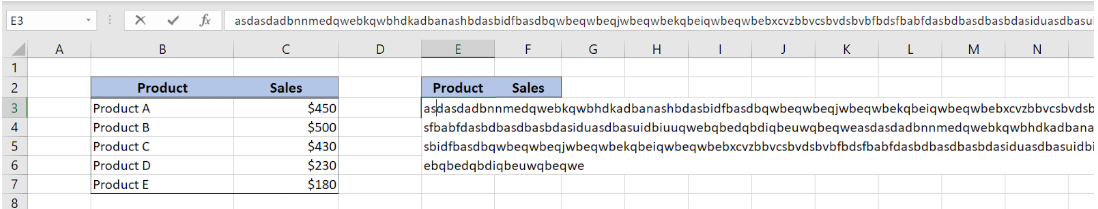Figure 1. The lookup value exceeding 255 characters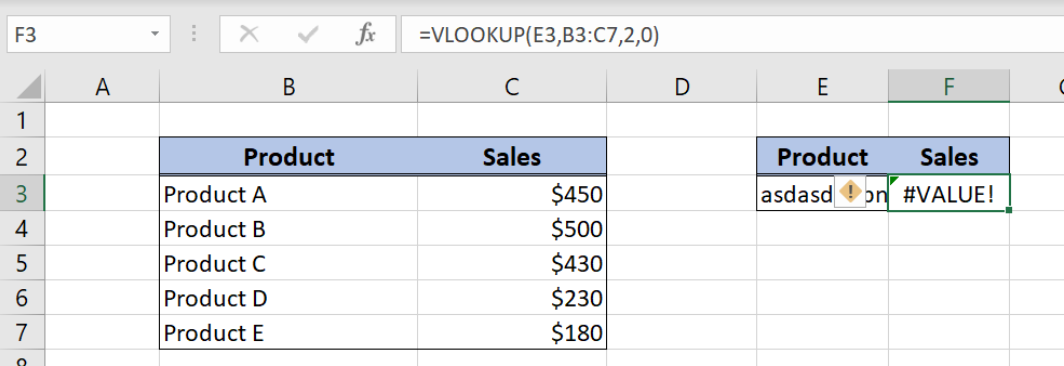Figure 2. #VALUE! Error as a result of the VLOOKUP function

The lookup value in the cell E3 is longer than 255 characters. As a result of the VLOOKUP function, we got #VALUE! error in the F3 cell.

## #N/A error in VLOOKUP function

The #N/A error in VLOOKUP appears when the result is not available. This can happen when the function can’t find a value which we are looking forward. We will show several examples when this error can occur.

• ### Typing errors or extra spaces in a value

There are some things that we should check in order to omit #N/A errors:

• possible typing errors in our lookup value
• leading or trailing spaces in our value

The leading or trailing spaces are sometimes tricky because we can’t see them in the beginning. These extra spaces mean that VLOOKUP will not find our value in a lookup table.

Here is the example of trailing spaces in cell B3: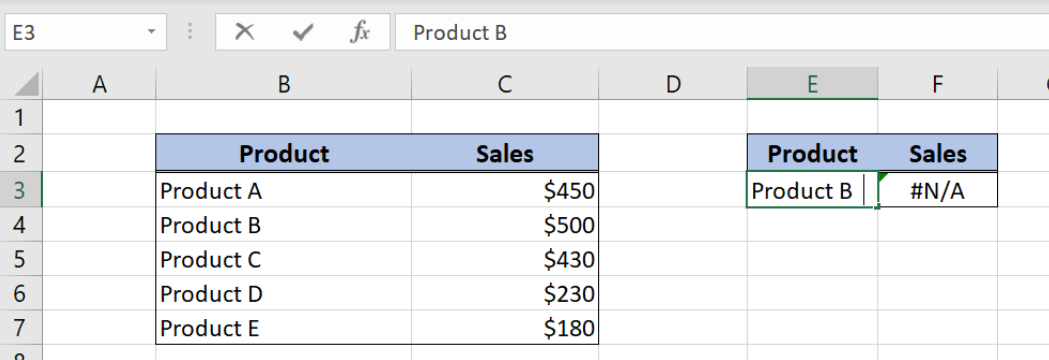Figure 3. #N/A value because of extra spaces in a value

As you can see, our lookup value in cell E3 has trailing spaces which we can’t see unless we click on it (“Product B   “). Because of the extra spaces, the value wasn’t found in the lookup table (B3:C7), and #N/A error is returned in the F3 cell.

One of the ways to solve this problem is to nest TRIM function into a VLOOKUP. The TRIM function clears all leading and trailing spaces from the string. By doing this, we will delete all spaces from the E3 cell and have value “Product B”. Here is the example: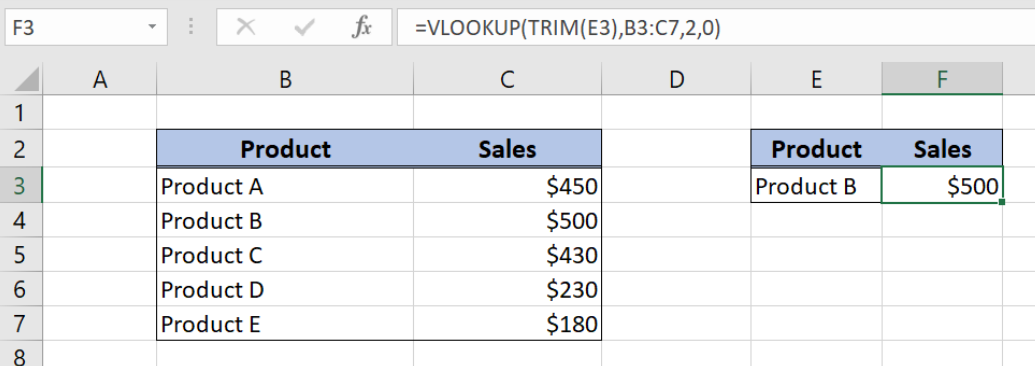Figure 4. TRIM function as a solution for #N/A error (extra spaces)

• ### Looking up in a column which is not the leftmost column

Another limitation of the VLOOKUP function is that the lookup column must be the leftmost column in a table. If we not follow this rule, we will get #N/A error.

Let’s look at the example to understand better this case: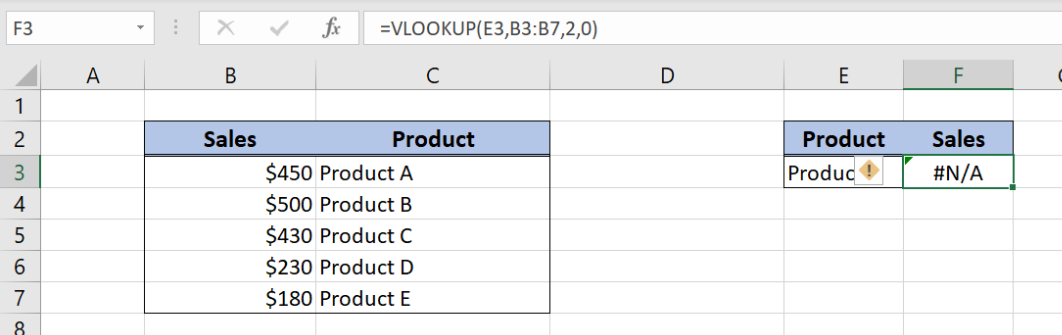Figure 5. Looking up in a column which is not the leftmost column

In this example, we want to find sales for Product B from the table B3:C7. However, the “Product” column is not the leftmost column in the table. As a result, we got #N/A error in the F3 cell, where we entered the VLOOKUP function.

Swap columns so that “Sales” is in column B, while “Product” is in column C. Now, we got the price \$500 for Product B in cell F3: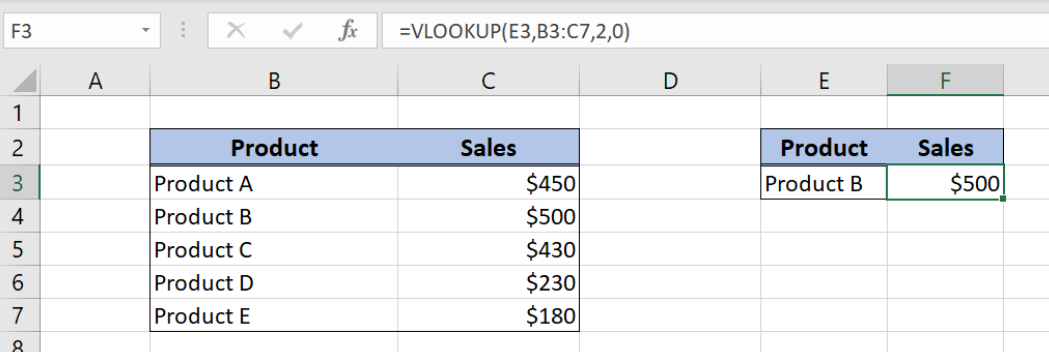Figure 6. The solution for #N/A error because of looking up in a column which is not the leftmost column

• ### Exact vs Approximate match

If we are using the exact match in VLOOKUP function, the parameter range_lookup should be set to 0. In this case, if the value is not found in a lookup table, the function will return #N/A error as a result.

On the other side, if we are using an approximate match, the parameter range_lookup should be set to 1. Then we can get #N/A error in two cases:

• If the lookup table is not sorted in ascending order
• If a value that we want to find is smaller than the smallest value in the lookup table.

Error appears in cell F3 because we didn’t set Product ID column values in ascending order: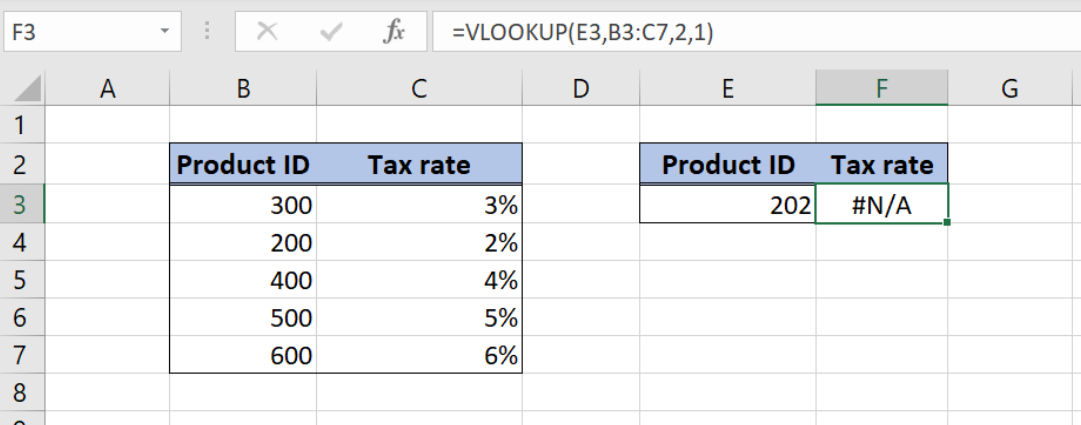Figure 7. #N/A error because Product ID Column is not sorted in ascending order

When we sort the values ascendingly in the column Product ID formula is working properly: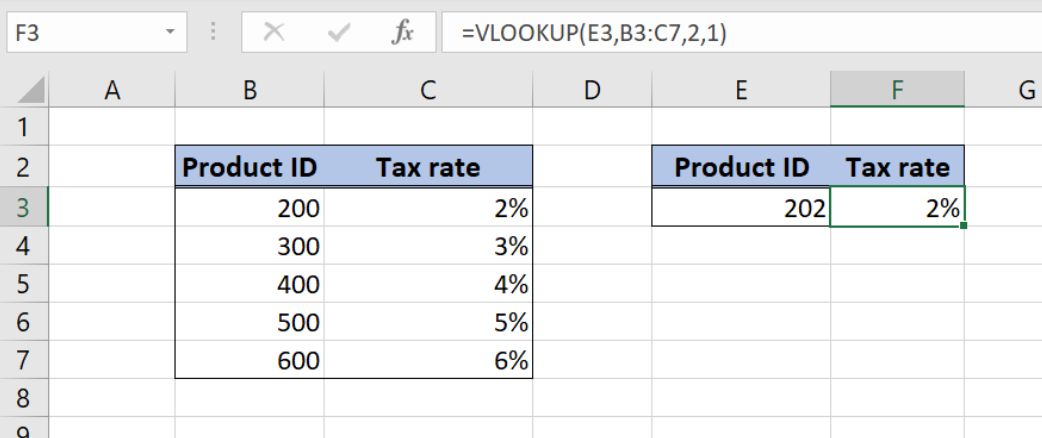Figure 8. Product ID Column sorted in ascending order

In the example below there is error in the cell F3 since the Product ID lookup value in the cell E3 is smaller than the smallest value in the column Product ID (Column B):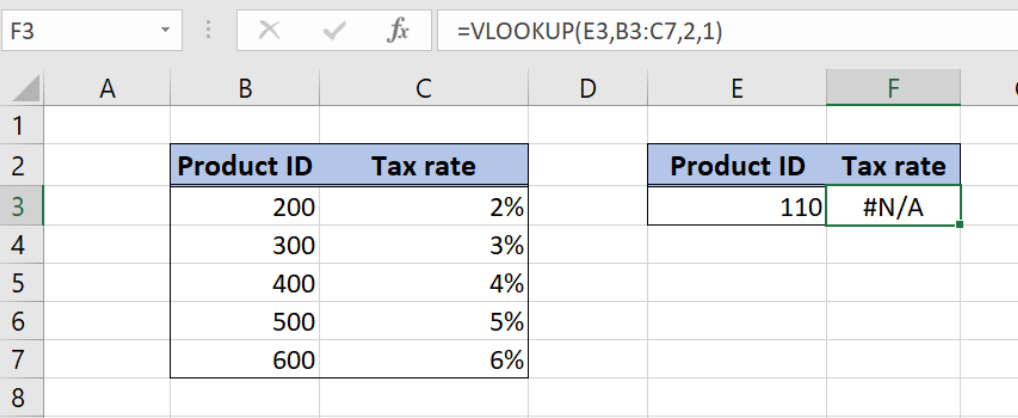Figure 9. Lookup value is smaller than the smallest value in the lookup table

## #NAME error in VLOOKUP function

This error appears when we misspell the function name. Therefore, this is the easiest error, since we just have to check the spelling of the formula name.

## Preventing errors display in VLOOKUP

Sometimes the value that we are trying to find isn’t in the data, which will cause #N/A error. When that happens, use IFERROR function to display more friendly error message or to prevent errors from displaying.

Above all, we can use IFERROR function with VLOOKUP. This function returns a certain value if there is an error. Therefore, we can set our error message, instead of #N/A or #VALUE!. The syntax looks like:

`=IFERROR(value, value_if_error)`

In our case, parameter value will be VLOOKUP function, while value_if_error will be our error message.

Here is the example. In column F3 we want to get Sales from column C, based on “Product B” form cell E3: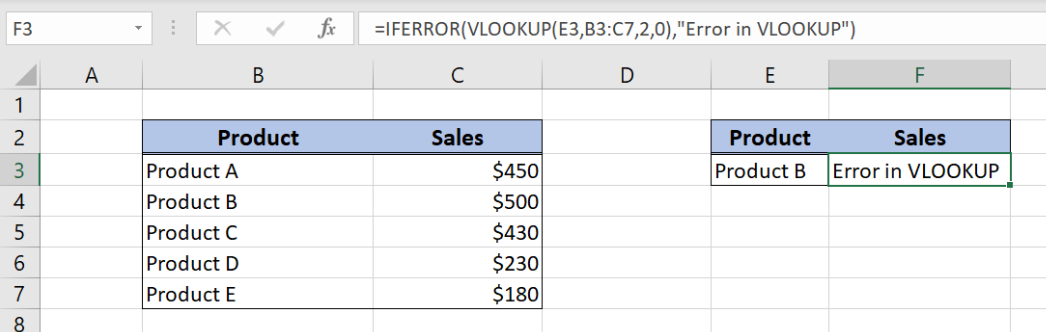Figure 10. IFERROR and VLOOKUP

The formula looks like:

`=IFERROR(VLOOKUP(E3,B3:C7,2,0), "Error in VLOOKUP")`

As you can see, we put VLOOKUP as the value parameter. On the other hand, we put our message “Error in VLOOKUP” in the value_if_error parameter. As Product B has extra spaces, the VLOOKUP returned an error, so the IFERROR returned our message in cell F3.

Most of the time, the problem you will need to solve will be more complex than a simple application of a formula or function. If you want to save hours of research and frustration, try our live Excelchat service! Our Excel Experts are available 24/7 to answer any Excel question you may have. We guarantee a connection within 30 seconds and a customized solution within 20 minutes.

### Did this post not answer your question? Get a solution from connecting with the expert.Another blog reader asked this question today on Excelchat:
Solution examplesI am trying to perform a vlookup or index match for a large data set. Normally I rely on vlookup but it is giving me data from the wrong column whatever I do. I have tried to move to vlookup and it is doing exactly the same thing. Do you have any tips?
Solved by O. W. in 27 minsI need help with Vlookup- am trying to copy a data from one sheet onto another matching the number but with a column
Solved by C. H. in 20 minsHi I'm trying to use fuzzy lookup. Not sure how it's working. I have two strings which are different ('Unemployment Benefits' vs 'Invalid pensioner'), yet getting a similarity index of 0.9474.
Solved by E. E. in 40 minsI have 2 sheets. One sheet with the demographics of contractors. eg. Company A, Hispanic, Male. In the second sheet I would like to be able to choose the company from a drop down menu (listing all companies) in column A and have columns B and C automatically populated with Hispanic and Male.
Solved by A. Y. in 34 minsGood Morning, I am trying to get parts from one column in an excel spread sheet to match up with a parts and cost column from a different excel spread sheet. (Not all parts on each spread sheet are the same)
Solved by S. L. in 49 mins## Subscribe to Excelchat.coAnother blog reader asked this question today on Excelchat: